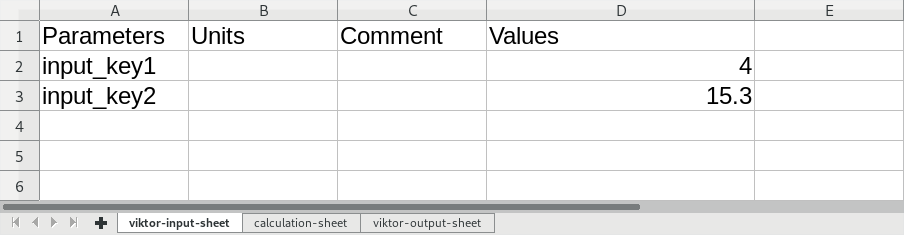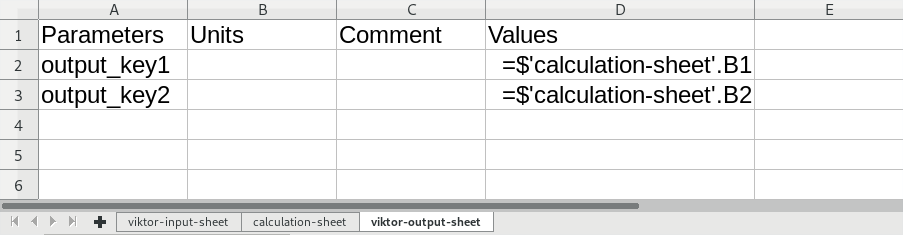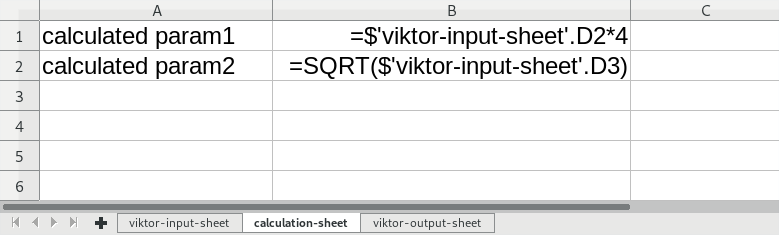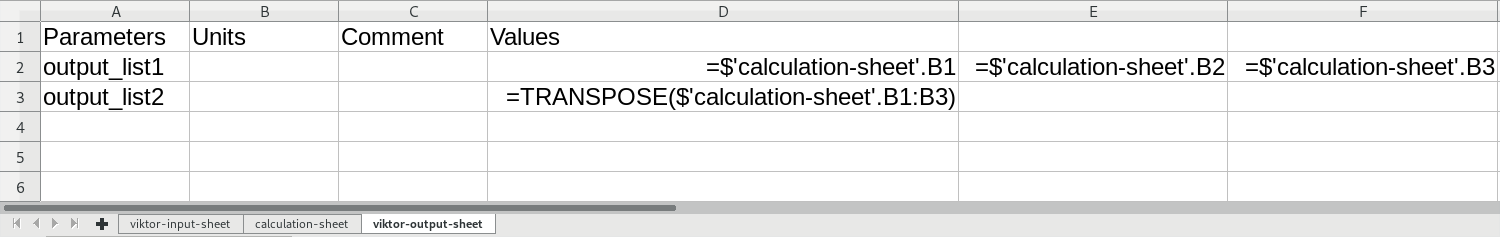tip

This guide is an informative piece to help you if you have questions about the Speadsheet calculation functionality. If you would like a more instructive lesson, take a look at the Spreadsheet calculator tutorial we have available.

caution

Services need to be mocked within the context of (automated) testing.

The `SpreadsheetCalculation` object can be used to utilize a spreadsheet without macros as an external calculator. If you want to use an Excel sheet with macros as a calculation tool, you can use VIKTOR's Excel integration.

## Calculation sheet set-up​

1. Add two sheets inside the Excel calculation document named "viktor-input-sheet" and "viktor-output-sheet".

caution

Note that these names must match in order for VIKTOR to find the correct sheet!

2. In the input and output sheets specify the following four columns:

• A1 = Parameters,
• B1 = Unit,
• C1 = Comment,
• D1 = Values
3. In "viktor-input-sheet": add input identifiers in the "Parameters" column and optionally specify default values in the "Values" columnviktor-input-sheet example

1. Link to these values as a source for the calculation in the calculation sheet
2. In "viktor-output-sheet": add output identifiers in the "Parameters" columnviktor-output-sheet example

1. In "viktor-output-sheet": point to the relevant cell(s) in the calculation sheetcalculation sheet example

## Implementation in app​

1. Import `SpreadsheetCalculation` and `SpreadsheetCalculationInput` from the `viktor.external` package

2. Evaluate the spreadsheet and obtain a SpreadsheetResult with a dictionary of the `output_key` parameters with corresponding values

``from viktor.external.spreadsheet import SpreadsheetCalculation, SpreadsheetCalculationInputdef calculation_result(a, b):    input_list = [        SpreadsheetCalculationInput('input_key1', a),        SpreadsheetCalculationInput('input_key2', b)    ]    spreadsheet = File.from_path('calculation_sheet.xlsx')    sheet = SpreadsheetCalculation(spreadsheet, input_list)    result = sheet.evaluate()    return result.valuescalculation_values = calculation_result(3, 6)print(calculation_values['output_key1'])``

## Using lists as input or output​

To pass a list of values as input for the calculation spreadsheet, one can pass a (e.g. semicolon) separated string ("value1;value2;value3;...") using `SpreadsheetCalculationInput` and write code logic in the spreadsheet to 'parse' and use the values.

It is also possible to store a range of cells to a list as output. Simply link the cells in the columns behind "Values" to the desired cells using an array formula.output list example

In the example `output_list1` and `output_list2` will return the same range of results.

caution

Currently a list can have a maximum of 47 values. A temporary workaround to make use of longer lists is by using a `TEXTJOIN` function in the calculation sheet. This function returns a joined string of the results separated by a user specified separator (e.g. ";"). In the client code, this list can then be converted back to a list of the results by using the `split()` function.